## Trigonometry

We've learned about the Pythagorean Theorem, the geometric mean, and magical right triangles that would give Harry Potter a run for his money. In this chapter, we've finally reached the center of the Tootsie-Pop, the delicious creamy filling in the center of every éclair.

Bon appétit, because it's time for trigo-nom-nom-nom-etry!

Trigonometry is a fancy term for triangle measurement. More specifically, it relates the angle measurements of triangles to the lengths of their sides. It's a big scary word, but the actual concepts aren't too complicated as long as you keep in mind everything we've talked about so far.

• ### Trig Ratios

We organize our trigonometric measurements by comparing the sides of a right triangle, creating three trigonometric ratios: sine, cosine, and tangent.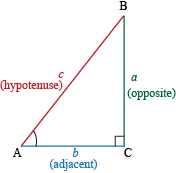Looking at ∠A, we can see clearly which side is adjacent, which is opposite, and which is the hypotenuse. Using these terms, we can define our three basic trigonometric ratios.

sin(A) = opposite / hypotenuse = a / h
cos(A) = adjacent / hypotenuse = b / h
tan(A) = opposite / adjacent = a / b

An easy way to remember which ratio is which is to just remember what someone who stubbed their foot would do and say. They'd soak their toe and say, "Ahhh."Soak a toe, ahhh. SOHCAHTOA. Each letter stands for something:

Sine: Opposite over Hypotenuse

It's important to know that these ratios depend on angles, not side lengths. That means it doesn't matter if a triangle is the size of Godzilla or the new iPod nano. If its angles are the same, it'll have the same trigonometric ratios.

We could stare at these fractions all day, but the only surefire way to learn trigonometry inside and out is to practice. Are you ready for this?

### Sample Problem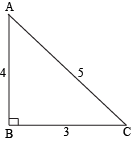We'll start simple and find the sine, cosine, and tangent for ∠A of this triangle.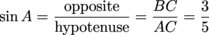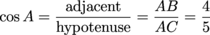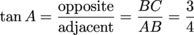That's all there is to it, putting one side over the other. For ∠C, though, these ratios would be different.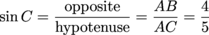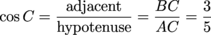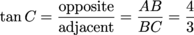Since these ratios never change for similar angles, we can use them to find the side lengths of similar triangles. That's kind of the whole point of trigonometry.

• ### Inverse Trig Ratios

If we have a right triangle, we only need two more pieces of information, and then we can calculate all the things.

1. We need to know a side length. Doesn't matter if it's the hypotenuse or one of the legs. Any ol' side length will do.
2. We need to know either another side length or one of the angle measurements. One that's not 90°, preferably.

### Sample Problem

For example, we have this triangle. If we want to know the length of the hypotenuse, finding a good angle and trig ratio to use should do the trick.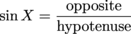Plug in what we know.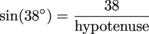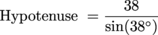Make sure your calculator is in degree mode, and then fire that baby up.

Hypotenuse ≈ 61.7

Armed with that knowledge, we can calculate the other leg of the triangle using the cosine.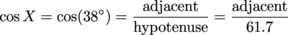However, using the leg we already have would be more accurate, so we'll stick with that.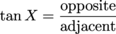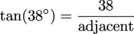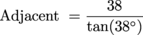Once again, we'll need a calculator.

Yippee. We now have all the side lengths and two out of the three angles. We can use the side lengths to figure out the remaining angle. Cosine hasn't been used yet, and we don't want it to feel left out.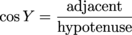This time, we're looking for Y. The adjacent side we plug in has to be adjacent to the angle we're using.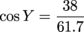cos Y = 0.616

Now what? Inverse cosine time.

That's right. Inverse cosine. If the cosine of B equals y, then the inverse cosine of y equals B. The same applies to sine and tangent, of course. These are called inverse trig functions, and they all look like this:

 Normal Trig Function Inverse Trig Function sin A = x sin-1(x) = A cos B = y cos-1(y) = B tan C = z tan-1(z) = C

The regular trig functions give us side lengths when we plug in an angle, so the inverse trig functions do the opposite: when we plug in a side length (like x, y, or z), we'll get an angle as our answer (A, B, or C).

Sometimes, these inverse trig functions are written with "arc" in front of their names. For instance, "sin-1(x)" turns into "arcsin(x)" and "cos-1(y)" becomes "arccos(y)." There's no difference. They mean the exact same thing.

Applying these inverse trig functions to our problem, if cos(Y) = 0.616, then cos-1(0.616) = Y. Surely our calculator can handle that. Again, make sure it's in degree mode.

cos-1(0.616) = Y
Y = 52°

A quick way to double-check that answer would be to add up all the angles in the triangle. All the angles in any triangle should always add up to 180°. Always.

X + Y + Z = 180°

We know that right angle Z is 90°, and we're given that angle X is 38°. We just calculated angle Y as 52°. We'll plug them in and cross our fingers.

38° + 52° + 90° = 180°
180° = 180°

Yessireebob. We got all our angles and all our side lengths using trigonometry.

### Sample ProblemCarl wants to relax in a hammock. Who doesn't, really? He's 6 feet away from one tree and 4 feet away from another. From where he's standing, he can see both trees are exactly 90° from each other. He wants us to find out the angles that the two trees make between each other and his own position. (Don't make him do it! He's on vacation!)

Relative to Tree A, we know the opposite and adjacent sides. If we're looking for trig ratios to use (which, hint-hint, we are), tangent would be a good choice.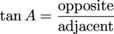Since we're looking for the angle, we should use the inverse tangent.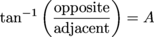Relative to Tree A, the opposite side is 4 feet and the adjacent side is 6 feet.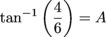A ≈ 33.7°

Now for Tree B.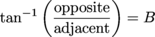Notice that relative to Tree B, the sides are switched. The opposite side is now 6 feet, and the adjacent side is now 4 feet.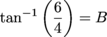B ≈ 56.3°

Carl appreciates our hard work, but he says that won't help much. He needs to know how long his hammock should be to hang it between the two trees. That means we need to figure out the distance between Tree A and Tree B.

We could use the Pythagorean Theorem, but why cramp our style? Trigonometry has served us well, so let's keep at it. We can use either the sine or cosine of either Tree A or B. Any one of those four should give us the same value for the hypotenuse.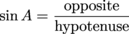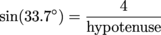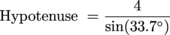Hypotenuse ≈ 7.2 feet

Carl's hammock should be at least 7.2 feet long. That's a nice, big hammock.

• ### Sine, Cosine, and Angles

It's also important to know the relationship between sine and cosine. We don't mean to pry into their personal lives, but it really is crucial. In order to find out what their relationship is, exactly, we can do a few calculations.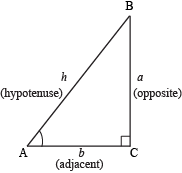We already know that sin A = ah and cos A = bh but what about ∠B? Remembering SOHCAHTOA, we can see that sin B = bh and cos B = ah. Was that the sound of the earth gasping? Yes, that's right.

sin A = cos B
cos A = sin B

Wait. It gets better. We know that there are 180° in a triangle, but the 90° takes half of that out of the equation. That means A + B = 90°. Using that relationship, we can clarify our sine-cosine relationship.

sin A = cos(90° – A)
cos A = sin(90° – A)

This means that sine and cosine are complementary trig functions (because the angles are complementary). Like the two main characters in any Nicholas Sparks novel, they complete each other.

Also relevant to this chapter are angles of elevation and depression. They both involve looking at cats. Awww.Angles of elevation are the angles from a horizontal to an upward line of sight, and angles of depression are from a horizontal to a downward line of sight. Like looking at a cat on top of a bookcase or sunbathing on the floor.